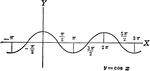### Cosine Curve y=cos x

Cosine curve plotted from negative pi to 2 pi. Graph of y=cos x.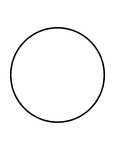### Flashcard of a Circle

A flashcard featuring an illustration of a Circle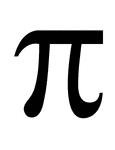### Flashcard of a math symbol for Pi

A flashcard featuring a math symbol for Pi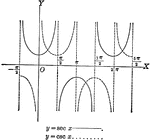### Secant and Cosecant Curves, y=sec x and y=csc x

Secant and Cosecant curves plotted from negative pi to 2 pi. Graph of y=sec x and y=csc x.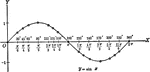### Sine Curve y=sin x

Sine curve plotted from 0 to 2 pi. Graph of y=sin x.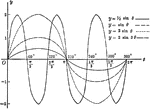### Sine Curves y= sin Ǝ, y= 1/2 sin Ǝ, y=2 sin Ǝ, y= 2 sin 3Ǝ

Sine curves of varying frequency and amplitude plotted from 0 to 2 pi. Graph of y= sin θ, y= 1/2…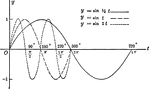### Sine Curves y= sin t, y= r sin1/2t, y= r sin 2t

Sine curves of varying frequency plotted from 0 to 2 pi. Graph of y= sin t, y= r sin1/2t, y=r sin 2t.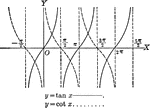### Tangent and Cotangent Curves, y=tan x and y=cot x

Tangent and Cotangent curves plotted from negative pi to 2 pi. Graph of y=tan x and y=cot x.